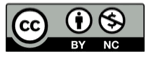# 笛卡尔叶形线

2018-07-01## 叶形线方程

$$r={{3a\sin \theta \cos \theta} \over {\sin^3 \theta + \cos^3 \theta}}, 0<\theta<\pi$$

## 参数的变化

$a$ 值取 $\{1,3,5,7,9,11\}$ 时，曲线的变化趋势

## 精彩评论